# Fundamentals of Physics - math problems

#### Number of problems found: 275

• Day trip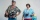A group of tourists went on a day trip. For two hours they walked at a speed of 6 km/h, for another hour they climbed a steep hill at a speed of 2 km/h, spent an hour and a half at lunch, then in an hour they inspected the castle garden at a free step at
• The temperature 13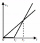The temperature in Toronto at noon during a winter day measured 4°C. The temperature started dropping 2° every hour. Which inequality can be used to find the number of hours, x, after which the temperature will measure below -3°C?
• The oscillation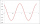The oscillation of a mechanical oscillator can be used to measure weight. A body of unknown weight is suspended on a spring with a stiffness of 20 N times m. The resulting oscillator oscillates with a frequency of 1.6 Hz. Find the weight of the body.
• Direction indicatorAs that which the electrical voltage is connected to the lamp direction indicator 7400 mOhm resistance when current of 1.6 A passes through it?
• Frequency of oscillation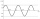A weight of 1 kg was suspended from the spring and the spring was extended by 1.5 cm. Determine the frequency of the natural oscillation of the resulting oscillator.
• Dynamometer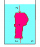What is the volume of a body that stretches a dynamometer in air, on which it is suspended by a force of 2.5 N, and if it is immersed in alcohol with a density of 800 kg/m ^ 3, does it tension the dynamometer with a force of 1.3 N?
• Now isNow is 5°C morning. What will the temperature be if i. It rises by 12°C ii. It falls by 15°C
• Above water surface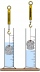If we remove the stone from the water, we apply a force of 120N. How much force will we have to exert if we move the stone above the water surface? The density of the stone is 5000 kg/m ^ 3.
• What power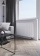What power should the radiator have in a room 5x4 meters with a height of 3 meters? Hint: Calculate 35 watts per meter ^ 3 in case you need 22 degrees in the room but you need about 24 degrees in the bathroom. It's an old heating cheat, but it will defini
• Two carsTwo cars (trucks and passenger) drove simultaneously from two places 50 km away. If they go against each other, they will meet in 30 minutes. If they follow each other in the same direction, the car will catch up with the truck in 2.5 hours. What is the s
• ThereThere is 5 l of water with a temperature of 20°C in the bucket. We add 3 liters of hot water. The resulting temperature will be 30°C. What was the temperature of the hot water?
• Two pendulums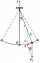In 24 s, the first pendulum performed 12 oscillations and the second 16 oscillations. Determine the periods and frequencies of both pendulums.
• Thermometer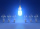Immerse a thermometer with a heat capacity of 2.0 J. K-1 in water weighing 67.0 grams. Before immersion in water, the thermometer showed a temperature of 17.8 degrees Celsius. After reaching equilibrium, the temperature is 32.4 degrees. What was the water
• Latent heat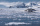How much heat is needed to take from 100 g of water at 20°C to cool to ice at -18°C? Mass heat capacity c (ice) = 21kJ/kg / °C; c (water) = 4.19 KJ/kg / °C and the mass group heat of solidification of water is l = 334kJ/kg
• Heat transferWe placed a lead object weighing 0.4 kg and 250°C in 0.4 L water. What was the initial temperature of water t2 if the object's temperature and the water after reaching equilibrium was 35°C? We assume that the heat exchange occurred only between the lead o
• The output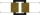The output voltage of the transformer is 880 V. The secondary coil has 1200 turns. Find the voltage to which the primary coil is connected and how many turns it has if a current of 1 A flows through it. Transformation ratio k = 4. What current flows throu
• The transformerThe primary coil of the transformer has 1100 turns and is connected to a voltage of 220 V. How many turns does the secondary coil have when it has a voltage of 55 V? Determine the transformation ratio and decide what the transformation is.
• The carThe car weight 1280 kg, increased its speed from 7.3 m/s to 63 km/h on a track of 37.2 m. What force did the car engine have to exert?
• Two boys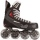Two boys weighing 60kg and 90kg move on roller skates and are attracted to each other by a rope. One boy exerts a force of 15N. What force does the other boy exert? What accelerations will the boys gain? Do not consider friction or air resistance.
• Tarzan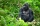Tarzan, weighing m = 90 kg, tries to cross the river by swinging with a vine hanging from a tree leaning over the river. Liana has a length l = 12 m. The speed of the Tarzan in the lowest swing position is v = 8 m/s. Tarzan doesn't know that the liana wil

Do you have an exciting math question or word problem that you can't solve? Ask a question or post a math problem, and we can try to solve it.

We will send a solution to your e-mail address. Solved examples are also published here. Please enter the e-mail correctly and check whether you don't have a full mailbox.

Please do not submit problems from current active competitions such as Mathematical Olympiad, correspondence seminars etc...